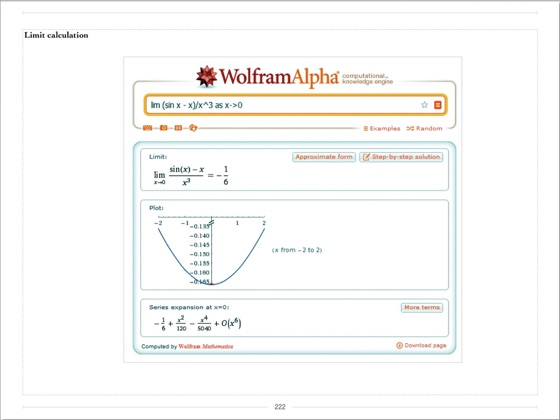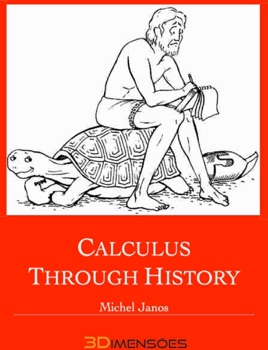• 4,49 €

## Descrizione dell’editore

The ideas of Calculus are a basic part of our culture; they have shaped how we perceive the world and how we perceive our place in the world. To understand how that is true of calculus, we must put Calculus into a historical perspective; we must contrast the world before Calculus with the world after Calculus.

Calculus is one of the most widely useful, if not the most widely useful, mathematical tool for understanding the real world. And yet, the development of Calculus required a willingness to use ideas, such as infinitesimals and limits, that are idealized and do not exist in the real world.

It is known that Newton and Leibniz invented Calculus in the 17th century. It turns out that a lot of the ingredients of Calculus were known before Newton and Leibniz. For other side it took over a century after Newton and Leibniz for Calculus to be worked out properly.

Calculus as we know it today was the result of a long evolution, not a revolution, though Newton and Leibniz were certainly the central figures.

In this book we follow the development of mathematics, through:

o Ancient Mathematics
o Pioneers of Modern Calculus
o Analysis
o Limits
o Newton and Leibniz
o Modern Calculus

This book contains animations and videos, and was produced with modern e-book resources.

•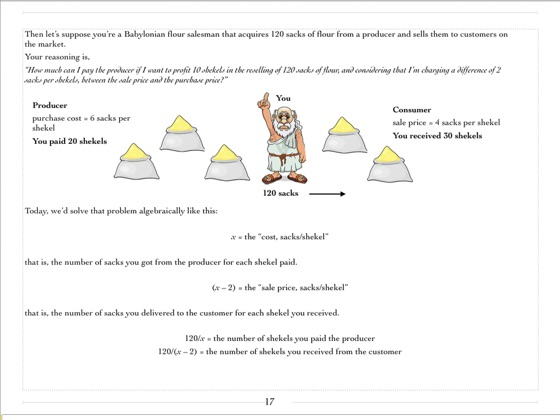•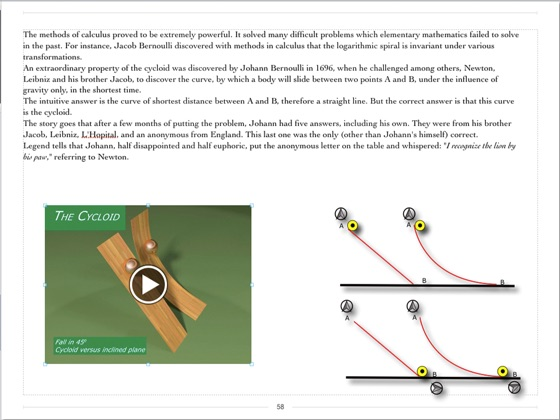•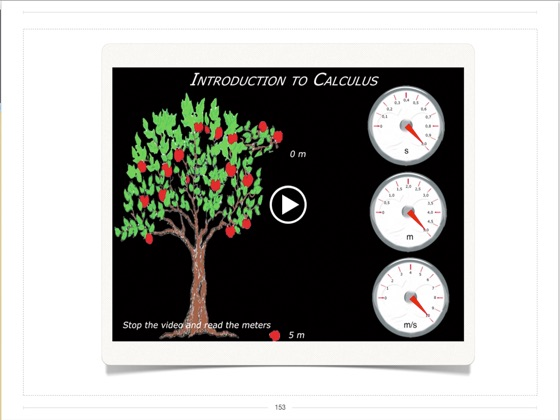•